Untitled24 (2)

# MNIST HANDWRITTEN DIGIT CLASSIFICATION�

MNIST handwritten digit classification is one of the most popular and fundamental deep learning problem. MNIST stands for Modified National Institute of Standards and Technology dataset. In this article, we will use Convolutional Neural Network(CNN) to form MNIST model which recognizes digit.

It is a dataset which consists of 28 X 28 pixels grayscale images of handwritten single digits between 0 and 9 both inclusive

In [ ]:
import tensorflow as tf
print(tf.__version__)         # To check tensorflow version

2.3.0


Make sure that you are using tensorflow 2.x i.e, 2.0.0 or above

In [ ]:
import pandas as pd
import numpy as np
import matplotlib.pyplot as plt


Each pixel is characterized by its (x, y) coordinates and its value. Digital images are characterized by matrix size, pixel depth and resolution. The matrix size is determined from the number of the columns (m) and the number of rows (n) of the image matrix (m � n).

2D or grayscale images are represented as (m, n). It consists of single channel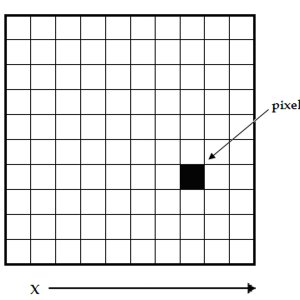whereas 3D images are represented as (m, n, 3) where 3 is the number of channels which is reprsented by RGB(Red Green Blue) colour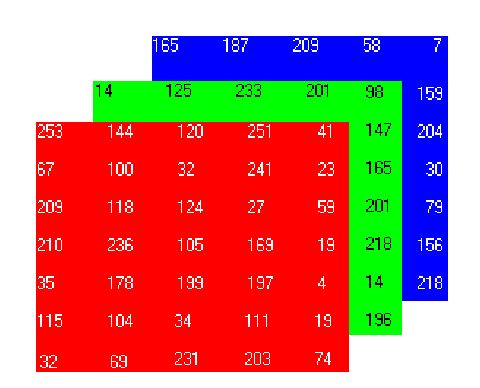MNIST dataset consists of 42000 training and 28000 testing grayscale images. Download dataset from the following link https://www.kaggle.com/c/digit-recognizer/data

This dataset is present in the CSV format so preprocess it to convert into matrix form which would represent image. Eaxh row of the dataset represents single image with corresponding label. We want each image of shape 28X28 pixels.

In [ ]:
# we want 28X28 pixel image
pixels = 28

In [ ]:
def line_2_img(img_dataset, csv_file):

# iterate through each rows of the csv file
for idx,line in enumerate(csv_file.iterrows()):

# getting single row
img = csv_file.iloc[idx]

# converting row into numpy array
img = np.array(img)

# converting 1D numpy array into 2D numpy array
img= np.array_split(img, 28)

# string into final dataset at corresponding index
img_dataset[idx, :, :] = img

return img_dataset

In [ ]:
def get_file(train_file, test_file):

# training dataset label
train_label = train_img_df['label']

# dropping label from tarining dataframe because we have created seperate dataframe for that
train_img_df.drop('label', axis=1, inplace=True)

# length of train dataset
train_length = train_img_df.shape

# creating numpy array for training image datatset initially filled with zero
train_img = np.zeros((train_length, 28, 28))

# length of test dataset
test_length = test_img_df.shape

# creating numpy array for testing image datatset initially filled with zero
test_img = np.zeros((test_length, 28, 28))

# getting training image dataset into the array format
train_img = line_2_img(train_img, train_img_df)

# getting test image dataset into the array format
test_img = line_2_img(test_img, test_img_df)

return train_img, train_label, test_img

In [ ]:
# training CSV dataset path
train_dir = '/content/gdrive/My Drive/digit-recognizer/train_digit.csv'

# testing CSV dataset path
test_dir = '/content/gdrive/My Drive/digit-recognizer/test_digit.csv'

In [ ]:
# converting CSV file into 2D image dataset
train, train_label, test = get_file(train_dir, test_dir)

In [ ]:
print('Train : {},  Test : {},  Training_Label : {}'.format(train.shape, test.shape, train_label.shape))

Train : (42000, 28, 28),  Test : (28000, 28, 28),  Training_Label : (42000,)


Given below are some of the training images with corresponding label

In [ ]:
# printing few training images with corresponding label
fig = plt.figure(figsize=(8, 8))

columns = 4
rows = 4
axes = []

# printing 16 training images
for i in range(1, columns*rows +1):
idx = np.random.randint(1, 100)
img = train[idx]
subplot_title = train_label[idx]
axes[-1].set_title(subplot_title)
plt.imshow(img, interpolation='nearest')
plt.axis('off')

plt.show()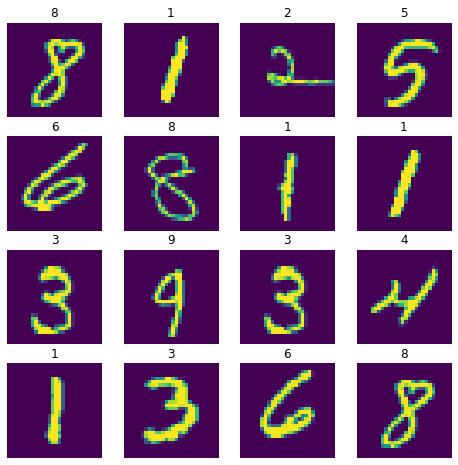In [ ]:
# plotting number of labels of each class
import seaborn as sns

train_lbl = pd.DataFrame(train_label)
train_lbl.columns = ['label']
sns.countplot(x = "label", data = train_lbl)
fig = plt.gcf()
fig.set_size_inches(10, 8)
plt.xlabel("Class")
plt.ylabel("Total Count")
plt.show()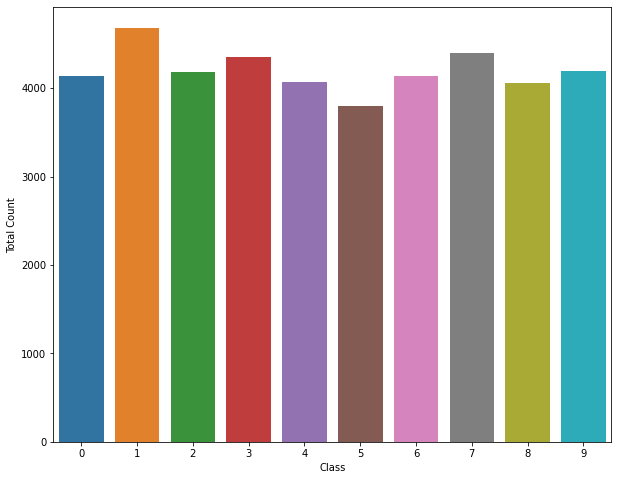In [ ]:
train_label = np.array(train_label)

# adding extra dimension to train and test image this extra dimension represents number of channel of grayscale image
train = np.expand_dims(train, axis=3)
test = np.expand_dims(test, axis=3)

In [ ]:
# converting 1D array to 2D array
train_label = np.expand_dims(train_label, axis=1)


Dividing train dataset into train and vaildation set of size ratio 90:10

In [ ]:
train_size = int(train.shape * 0.9)

train_img, valid_img = train[ : train_size], train[train_size : ]
train_label, valid_label = train_label[ : train_size], train_label[train_size : ]


Generate batches of tensor image data with real-time data augmentation.

In [ ]:
from tensorflow.keras.preprocessing.image import ImageDataGenerator

In [ ]:
train_datagen = ImageDataGenerator(
rescale=1./255,                       # normalizing each pixels of image
rotation_range=30,                    #  Integer Degree range for random rotations.
width_shift_range=0.2,
height_shift_range=0.2,
shear_range=0.2,                      # Shear Intensity (Shear angle in counter-clockwise direction in degrees)
zoom_range=0.2,                       # Range for random zoom
fill_mode='nearest',                  #  Points outside the boundaries of the input are filled
horizontal_flip=False,                #  Boolean randomly flip inputs horizontally.
vertical_flip = False                 #  Boolean randomly flip inputs vertically
)

In [ ]:
validation_datagen = ImageDataGenerator(
rescale=1./255
)


Training CNN model to classify each image as one of the label from 0 - 9

In [ ]:
# Loading Libraries
from tensorflow.keras import Sequential
from tensorflow.keras.layers import Conv2D, MaxPooling2D, Flatten,Dropout,Dense
from tensorflow.keras.optimizers import RMSprop

In [ ]:
# building model
model = tf.keras.Sequential([
Conv2D(64, (3,3), activation='relu', input_shape=(28, 28, 1),  padding='same'),
MaxPooling2D(2, 2),
Dropout(0.4),

Conv2D(128, (3, 3), activation='relu'),
MaxPooling2D(2, 2),
Dropout(0.4),

Flatten(),

Dense(512, activation='relu'),
Dense(256, activation='relu'),
Dense(128, activation='relu'),
Dense(10, activation='softmax')
])

In [ ]:
# model compile
model.compile(optimizer = RMSprop(lr=0.0001),
loss = 'sparse_categorical_crossentropy',
metrics = ['acc'])

In [ ]:
# model architecture summary
model.summary()

Model: "sequential"
_________________________________________________________________
Layer (type)                 Output Shape              Param #
=================================================================
conv2d (Conv2D)              (None, 28, 28, 64)        640
_________________________________________________________________
conv2d_1 (Conv2D)            (None, 28, 28, 64)        36928
_________________________________________________________________
max_pooling2d (MaxPooling2D) (None, 14, 14, 64)        0
_________________________________________________________________
dropout (Dropout)            (None, 14, 14, 64)        0
_________________________________________________________________
conv2d_2 (Conv2D)            (None, 12, 12, 128)       73856
_________________________________________________________________
conv2d_3 (Conv2D)            (None, 12, 12, 128)       147584
_________________________________________________________________
max_pooling2d_1 (MaxPooling2 (None, 6, 6, 128)         0
_________________________________________________________________
dropout_1 (Dropout)          (None, 6, 6, 128)         0
_________________________________________________________________
flatten (Flatten)            (None, 4608)              0
_________________________________________________________________
dense (Dense)                (None, 512)               2359808
_________________________________________________________________
dense_1 (Dense)              (None, 256)               131328
_________________________________________________________________
dense_2 (Dense)              (None, 128)               32896
_________________________________________________________________
dense_3 (Dense)              (None, 10)                1290
=================================================================
Total params: 2,784,330
Trainable params: 2,784,330
Non-trainable params: 0
_________________________________________________________________

In [ ]:
# training CNN model over 15 epochs
train_generator = train_datagen.flow(train_img, train_label, batch_size=32)
validation_generator = validation_datagen.flow(valid_img, valid_label, batch_size=32)

history = model.fit_generator(train_generator,
epochs=15,
verbose=1,
validation_data=validation_generator)

WARNING:tensorflow:From <ipython-input-26-6895fe6d3c88>:8: Model.fit_generator (from tensorflow.python.keras.engine.training) is deprecated and will be removed in a future version.
Instructions for updating:
Please use Model.fit, which supports generators.
Epoch 1/15
1182/1182 [==============================] - 14s 12ms/step - loss: 1.2145 - acc: 0.1407 - val_loss: 0.2311 - val_acc: 0.1131
Epoch 2/15
1182/1182 [==============================] - 13s 11ms/step - loss: 0.4801 - acc: 0.0966 - val_loss: 0.1505 - val_acc: 0.1088
Epoch 3/15
1182/1182 [==============================] - 14s 11ms/step - loss: 0.3138 - acc: 0.0975 - val_loss: 0.0860 - val_acc: 0.1081
Epoch 4/15
1182/1182 [==============================] - 14s 12ms/step - loss: 0.2418 - acc: 0.0973 - val_loss: 0.0653 - val_acc: 0.1083
Epoch 5/15
1182/1182 [==============================] - 14s 12ms/step - loss: 0.1896 - acc: 0.0976 - val_loss: 0.0627 - val_acc: 0.1074
Epoch 6/15
1182/1182 [==============================] - 13s 11ms/step - loss: 0.1637 - acc: 0.0976 - val_loss: 0.0472 - val_acc: 0.1083
Epoch 7/15
1182/1182 [==============================] - 13s 11ms/step - loss: 0.1456 - acc: 0.0975 - val_loss: 0.0446 - val_acc: 0.1083
Epoch 8/15
1182/1182 [==============================] - 13s 11ms/step - loss: 0.1283 - acc: 0.0974 - val_loss: 0.0684 - val_acc: 0.1043
Epoch 9/15
1182/1182 [==============================] - 13s 11ms/step - loss: 0.1228 - acc: 0.0979 - val_loss: 0.0386 - val_acc: 0.1093
Epoch 10/15
1182/1182 [==============================] - 14s 12ms/step - loss: 0.1102 - acc: 0.0978 - val_loss: 0.0426 - val_acc: 0.1081
Epoch 11/15
1182/1182 [==============================] - 13s 11ms/step - loss: 0.1054 - acc: 0.0976 - val_loss: 0.0340 - val_acc: 0.1074
Epoch 12/15
1182/1182 [==============================] - 13s 11ms/step - loss: 0.0992 - acc: 0.0973 - val_loss: 0.0424 - val_acc: 0.1083
Epoch 13/15
1182/1182 [==============================] - 14s 11ms/step - loss: 0.0948 - acc: 0.0977 - val_loss: 0.0375 - val_acc: 0.1071
Epoch 14/15
1182/1182 [==============================] - 14s 11ms/step - loss: 0.0946 - acc: 0.0979 - val_loss: 0.0318 - val_acc: 0.1093
Epoch 15/15
1182/1182 [==============================] - 13s 11ms/step - loss: 0.0908 - acc: 0.0976 - val_loss: 0.0364 - val_acc: 0.1079


Plotting accuracy vs epochs to visualize model performance

In [ ]:
plt.plot(history.history['acc'])
plt.plot(history.history['val_acc'])
plt.title('model accuracy')
plt.ylabel('accuracy')
plt.xlabel('epoch')
plt.legend(['train', 'valid'], loc='upper left')
plt.show()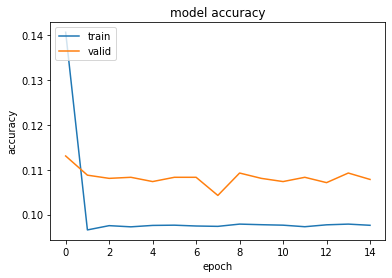Plotting loss vs epochs to visualize model performance

In [ ]:
plt.plot(history.history['loss'])
plt.plot(history.history['val_loss'])
plt.title('model loss')
plt.ylabel('loss')
plt.xlabel('epoch')
plt.legend(['train', 'valid'], loc='upper left')
plt.show()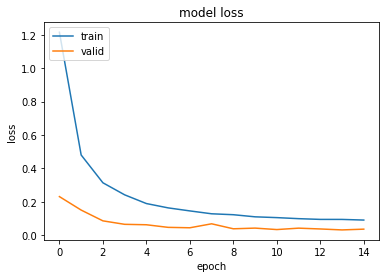Evaluating given model over test dataset gives 98.89% accuracy

Visualizing intermediate activations of each layer of CNN model

In [ ]:
# visualizing each layer output based on below example
img_tensor = test
img_tensor = img_tensor.reshape(28, 28)

plt.imshow(img_tensor)

Out[ ]:
<matplotlib.image.AxesImage at 0x7f5c1d2a6fd0>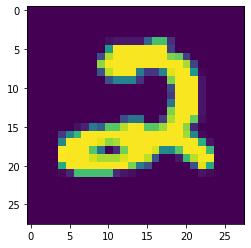In [ ]:
img_tensor = np.expand_dims(img_tensor, axis=0)

In [ ]:
from keras import models
# extracting output of each layer of model
layer_outputs = [layer.output for layer in model.layers]
activation_model = models.Model(inputs=model.input, outputs=layer_outputs)

activations = activation_model.predict(img_tensor)
print('Number of layers in the model : {}'.format(len(activations)))

Number of layers in the model : 13

In [ ]:
# name of each layer
layer_names = []

for layer in model.layers:
layer_names.append(layer.name)
print('Name of first 4 layers : {}'.format(layer_names[:4]))

Name of first 4 layers : ['conv2d', 'conv2d_1', 'max_pooling2d', 'dropout']


Activation of first layer

In [ ]:
# number of images displaying in each row
images_per_row = 16

# first layer activation
first_layer_activation = activations

# Number of features in the feature map
n_features = first_layer_activation.shape[-1]

#The feature map has shape (1, size, size, n_features).
size = first_layer_activation.shape

# Tiles the activation channels in this matrix
n_rows = n_features // images_per_row

display_grid = np.zeros((size * n_rows, images_per_row * size))

for row_number in range(n_rows):
for row in range(images_per_row):
feature_n = row_number * images_per_row + row
channel_image = first_layer_activation[0,:, :, feature_n]
channel_image -= channel_image.mean() # Post-processes the feature to make it visually palatable
channel_image /= channel_image.std()
channel_image *= 64
channel_image += 128

channel_image = np.clip(channel_image, 0, 255).astype('uint8')
display_grid[row_number * size : (row_number + 1) * size, # Displays the grid
row * size : (row + 1) * size ] = channel_image

scale = 1. / size
plt.figure(figsize=(scale * display_grid.shape,
scale * display_grid.shape))
plt.title(layer_names)
plt.grid(False)
plt.axis('off')
plt.imshow(display_grid, aspect='auto', cmap='viridis')

Out[ ]:
<matplotlib.image.AxesImage at 0x7f5c1cf7ed68>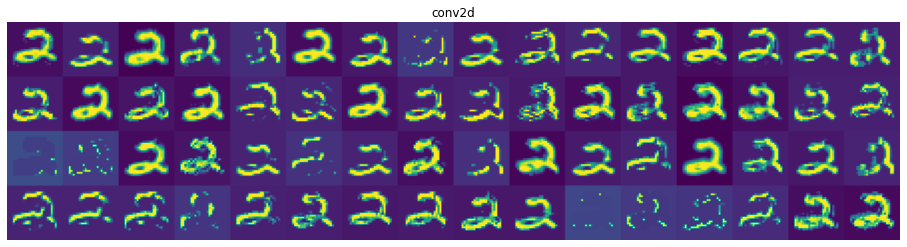Similarly, you can get activation of other layers too.

Browser Based Tensorflow.js model

install " !pip install tensorflowjs " if not already installed

In [ ]:
!pip install tensorflowjs

Collecting tensorflowjs
|%u2588%u2588%u2588%u2588%u2588%u2588%u2588%u2588%u2588%u2588%u2588%u2588%u2588%u2588%u2588%u2588%u2588%u2588%u2588%u2588%u2588%u2588%u2588%u2588%u2588%u2588%u2588%u2588%u2588%u2588%u2588%u2588| 71kB 4.7MB/s
Requirement already satisfied: tensorflow-hub<0.10,>=0.7.0 in /usr/local/lib/python3.6/dist-packages (from tensorflowjs) (0.9.0)
Requirement already satisfied: six<2,>=1.12.0 in /usr/local/lib/python3.6/dist-packages (from tensorflowjs) (1.15.0)
Requirement already satisfied: tensorflow<3,>=2.1.0 in /usr/local/lib/python3.6/dist-packages (from tensorflowjs) (2.3.0)
Requirement already satisfied: h5py<3,>=2.8.0 in /usr/local/lib/python3.6/dist-packages (from tensorflowjs) (2.10.0)
Requirement already satisfied: protobuf>=3.8.0 in /usr/local/lib/python3.6/dist-packages (from tensorflow-hub<0.10,>=0.7.0->tensorflowjs) (3.12.4)
Requirement already satisfied: numpy>=1.12.0 in /usr/local/lib/python3.6/dist-packages (from tensorflow-hub<0.10,>=0.7.0->tensorflowjs) (1.18.5)
Requirement already satisfied: wrapt>=1.11.1 in /usr/local/lib/python3.6/dist-packages (from tensorflow<3,>=2.1.0->tensorflowjs) (1.12.1)
Requirement already satisfied: wheel>=0.26 in /usr/local/lib/python3.6/dist-packages (from tensorflow<3,>=2.1.0->tensorflowjs) (0.35.1)
Requirement already satisfied: tensorboard<3,>=2.3.0 in /usr/local/lib/python3.6/dist-packages (from tensorflow<3,>=2.1.0->tensorflowjs) (2.3.0)
Requirement already satisfied: gast==0.3.3 in /usr/local/lib/python3.6/dist-packages (from tensorflow<3,>=2.1.0->tensorflowjs) (0.3.3)
Requirement already satisfied: opt-einsum>=2.3.2 in /usr/local/lib/python3.6/dist-packages (from tensorflow<3,>=2.1.0->tensorflowjs) (3.3.0)
Requirement already satisfied: astunparse==1.6.3 in /usr/local/lib/python3.6/dist-packages (from tensorflow<3,>=2.1.0->tensorflowjs) (1.6.3)
Requirement already satisfied: keras-preprocessing<1.2,>=1.1.1 in /usr/local/lib/python3.6/dist-packages (from tensorflow<3,>=2.1.0->tensorflowjs) (1.1.2)
Requirement already satisfied: scipy==1.4.1 in /usr/local/lib/python3.6/dist-packages (from tensorflow<3,>=2.1.0->tensorflowjs) (1.4.1)
Requirement already satisfied: grpcio>=1.8.6 in /usr/local/lib/python3.6/dist-packages (from tensorflow<3,>=2.1.0->tensorflowjs) (1.32.0)
Requirement already satisfied: absl-py>=0.7.0 in /usr/local/lib/python3.6/dist-packages (from tensorflow<3,>=2.1.0->tensorflowjs) (0.10.0)
Requirement already satisfied: termcolor>=1.1.0 in /usr/local/lib/python3.6/dist-packages (from tensorflow<3,>=2.1.0->tensorflowjs) (1.1.0)
Requirement already satisfied: tensorflow-estimator<2.4.0,>=2.3.0 in /usr/local/lib/python3.6/dist-packages (from tensorflow<3,>=2.1.0->tensorflowjs) (2.3.0)
Requirement already satisfied: setuptools in /usr/local/lib/python3.6/dist-packages (from protobuf>=3.8.0->tensorflow-hub<0.10,>=0.7.0->tensorflowjs) (50.3.0)
Requirement already satisfied: werkzeug>=0.11.15 in /usr/local/lib/python3.6/dist-packages (from tensorboard<3,>=2.3.0->tensorflow<3,>=2.1.0->tensorflowjs) (1.0.1)
Requirement already satisfied: requests<3,>=2.21.0 in /usr/local/lib/python3.6/dist-packages (from tensorboard<3,>=2.3.0->tensorflow<3,>=2.1.0->tensorflowjs) (2.23.0)
Requirement already satisfied: tensorboard-plugin-wit>=1.6.0 in /usr/local/lib/python3.6/dist-packages (from tensorboard<3,>=2.3.0->tensorflow<3,>=2.1.0->tensorflowjs) (1.7.0)
Requirement already satisfied: markdown>=2.6.8 in /usr/local/lib/python3.6/dist-packages (from tensorboard<3,>=2.3.0->tensorflow<3,>=2.1.0->tensorflowjs) (3.2.2)
Requirement already satisfied: certifi>=2017.4.17 in /usr/local/lib/python3.6/dist-packages (from requests<3,>=2.21.0->tensorboard<3,>=2.3.0->tensorflow<3,>=2.1.0->tensorflowjs) (2020.6.20)
Requirement already satisfied: chardet<4,>=3.0.2 in /usr/local/lib/python3.6/dist-packages (from requests<3,>=2.21.0->tensorboard<3,>=2.3.0->tensorflow<3,>=2.1.0->tensorflowjs) (3.0.4)
Requirement already satisfied: urllib3!=1.25.0,!=1.25.1,<1.26,>=1.21.1 in /usr/local/lib/python3.6/dist-packages (from requests<3,>=2.21.0->tensorboard<3,>=2.3.0->tensorflow<3,>=2.1.0->tensorflowjs) (1.24.3)
Requirement already satisfied: idna<3,>=2.5 in /usr/local/lib/python3.6/dist-packages (from requests<3,>=2.21.0->tensorboard<3,>=2.3.0->tensorflow<3,>=2.1.0->tensorflowjs) (2.10)
Requirement already satisfied: rsa<5,>=3.1.4; python_version >= "3" in /usr/local/lib/python3.6/dist-packages (from google-auth<2,>=1.6.3->tensorboard<3,>=2.3.0->tensorflow<3,>=2.1.0->tensorflowjs) (4.6)
Requirement already satisfied: importlib-metadata; python_version < "3.8" in /usr/local/lib/python3.6/dist-packages (from markdown>=2.6.8->tensorboard<3,>=2.3.0->tensorflow<3,>=2.1.0->tensorflowjs) (2.0.0)
Requirement already satisfied: pyasn1>=0.1.3 in /usr/local/lib/python3.6/dist-packages (from rsa<5,>=3.1.4; python_version >= "3"->google-auth<2,>=1.6.3->tensorboard<3,>=2.3.0->tensorflow<3,>=2.1.0->tensorflowjs) (0.4.8)
Requirement already satisfied: zipp>=0.5 in /usr/local/lib/python3.6/dist-packages (from importlib-metadata; python_version < "3.8"->markdown>=2.6.8->tensorboard<3,>=2.3.0->tensorflow<3,>=2.1.0->tensorflowjs) (3.2.0)
Installing collected packages: tensorflowjs
Successfully installed tensorflowjs-2.6.0

In [ ]:
import tensorflowjs as tfjs


Saving model into HDF5 format

In [ ]:
model.save('/content/gdrive/My Drive/digit-recognizer/model_updt.h5')


Converting HDF5 model into tensorflowjs model format. After conversion download the files from output directory

In [ ]:
# output directory
path = '/content/gdrive/My Drive/PROJECT'

tfjs.converters.save_keras_model(model, path)

/usr/local/lib/python3.6/dist-packages/tensorflowjs/converters/keras_h5_conversion.py:123: H5pyDeprecationWarning: The default file mode will change to 'r' (read-only) in h5py 3.0. To suppress this warning, pass the mode you need to h5py.File(), or set the global default h5.get_config().default_file_mode, or set the environment variable H5PY_DEFAULT_READONLY=1. Available modes are: 'r', 'r+', 'w', 'w-'/'x', 'a'. See the docs for details.
return h5py.File(h5file)


HTML file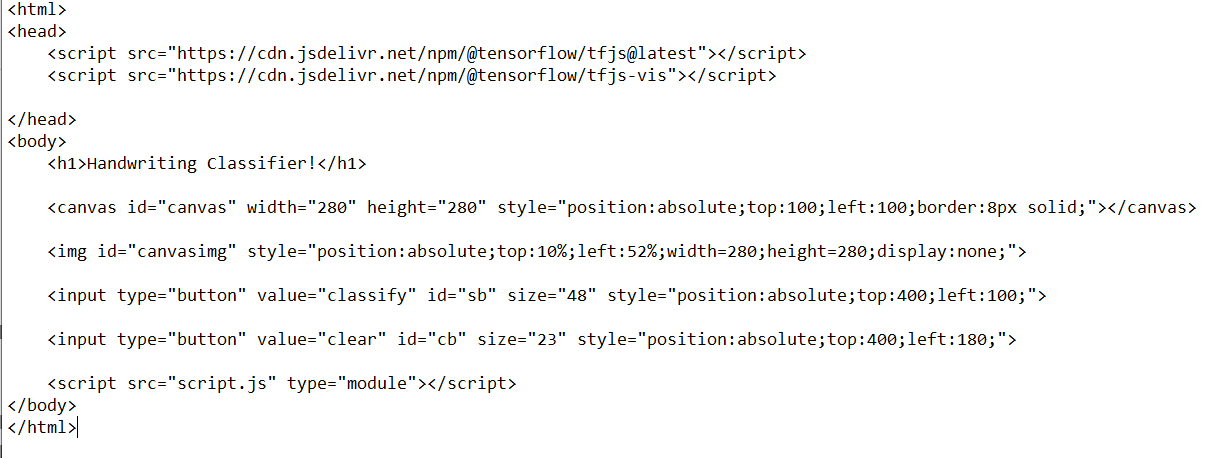JavaScript file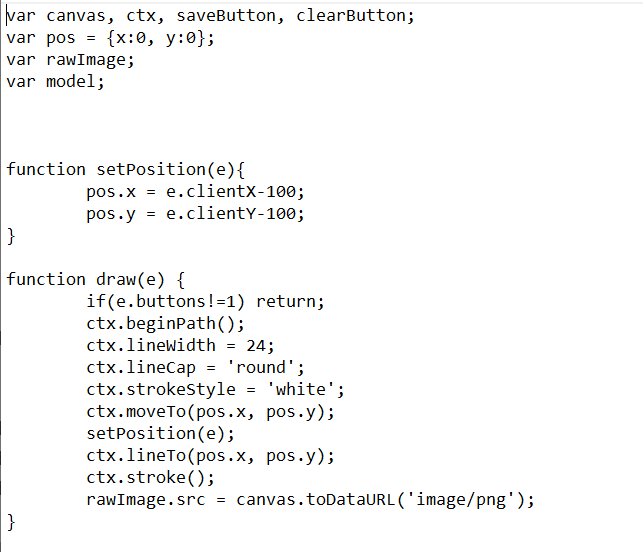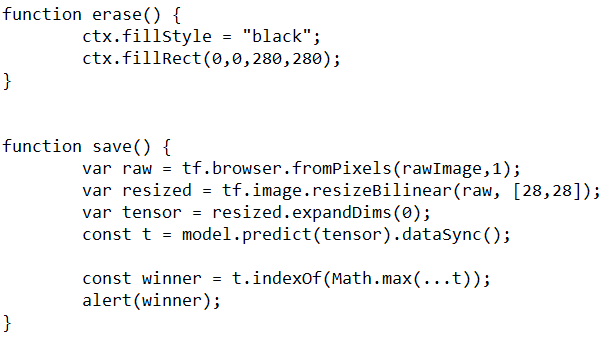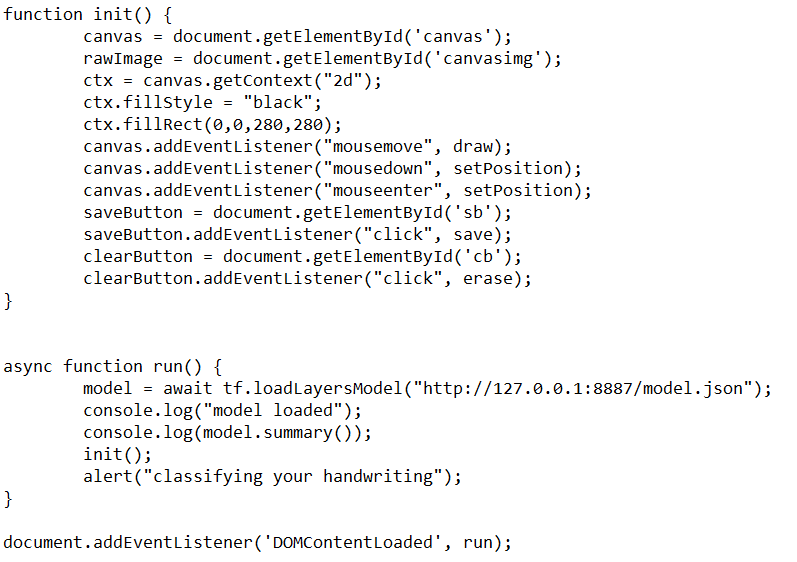After creating HTML and JavaScript put all your Files into same directory

Files

1. HTML file
2. JavaScript file
3. Saved model files

To run project install :

1. Chrome
2. Web Server for Chrome